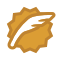# Uniform Generator

Time Limit: 2000/1000 MS (Java/Others)    Memory Limit: 65536/32768 K (Java/Others)
Total Submission(s): 14697    Accepted Submission(s): 5739

Problem Description
Computer simulations often require random numbers. One way to generate pseudo-random numbers is via a function of the form

seed(x+1) = [seed(x) + STEP] % MOD

where '%' is the modulus operator.

Such a function will generate pseudo-random numbers (seed) between 0 and MOD-1. One problem with functions of this form is that they will always generate the same pattern over and over. In order to minimize this effect, selecting the STEP and MOD values carefully can result in a uniform distribution of all values between (and including) 0 and MOD-1.

For example, if STEP = 3 and MOD = 5, the function will generate the series of pseudo-random numbers 0, 3, 1, 4, 2 in a repeating cycle. In this example, all of the numbers between and including 0 and MOD-1 will be generated every MOD iterations of the function. Note that by the nature of the function to generate the same seed(x+1) every time seed(x) occurs means that if a function will generate all the numbers between 0 and MOD-1, it will generate pseudo-random numbers uniformly with every MOD iterations.

If STEP = 15 and MOD = 20, the function generates the series 0, 15, 10, 5 (or any other repeating series if the initial seed is other than 0). This is a poor selection of STEP and MOD because no initial seed will generate all of the numbers from 0 and MOD-1.

Your program will determine if choices of STEP and MOD will generate a uniform distribution of pseudo-random numbers.

Input
Each line of input will contain a pair of integers for STEP and MOD in that order (1 <= STEP, MOD <= 100000).

Output
For each line of input, your program should print the STEP value right- justified in columns 1 through 10, the MOD value right-justified in columns 11 through 20 and either "Good Choice" or "Bad Choice" left-justified starting in column 25. The "Good Choice" message should be printed when the selection of STEP and MOD will generate all the numbers between and including 0 and MOD-1 when MOD numbers are generated. Otherwise, your program should print the message "Bad Choice". After each output test set, your program should print exactly one blank line.

Sample Input
3 5 15 20 63923 99999

Sample Output
3 5 Good Choice 15 20 Bad Choice 63923 99999 Good Choice

算法思想：

首先、写出判断互质数函数，即最大公约数为1 ；

然后； 没了

#include<iostream>
#include<cstdio>
using namespace std;

int gcd(int a, int b)
{
if( b == 0 )
{
return  a;
}
int t = a%b;
while(t)
{
a = b;
b = t;
t = a%b;
}
return  b;
}
int main()
{
int a, b;
while(cin>>a>>b)
{
if(gcd(a, b) == 1)
printf("%10d%10d    Good Choice\n\n", a, b);
else
}
return 0;
}


wangxiaomingCSDN认证博客专家 架构 Spring Boot Redis08-04354

#### hdu1014

05-14169

#### HoloLens2开发入门教程

05-01

02-1945万+

#### Java基础知识面试题（2020最新版）©️2020 CSDN 皮肤主题: Age of Ai 设计师: meimeiellie点击重新获取扫码支付1.余额是钱包充值的虚拟货币，按照1:1的比例进行支付金额的抵扣。
2.余额无法直接购买下载，可以购买VIP、C币套餐、付费专栏及课程。余额充值